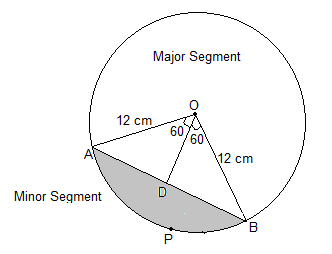# A chord of a circle of radius 12 cm subtends an angle of 120° at the centre. Find the area of the corresponding segment of the circle. (Use π = 3.14 and √3 = 1.73)Draw a perpendicular OD on chord AB and it will bisect chord AB.

Area of triangle=1/2*base*height

Area of segment=Area of the sector -the area of a triangle

The area of the minor sector = (θ/360°)×πr2

= (120/360)×(22/7)×122

= 150.72 cm2

From the ΔAOB,

∠ OAB = 180°-(90°+60°) = 30°

We know that OD bisects AB. So,

AB = 2×AD = 12√3 cm

Now, sin 30° = OD/OA

Or, ½ = OD/12

∴ OD = 6 cm

So, the area of ΔAOB = ½ × base × height

Here, base = AB = 12√3 and

Height = OD = 6

So, area of ΔAOB = ½×12√3×6 = 36√3 cm = 62.28 cm2

∴ Area of the corresponding Minor segment = Area of the Minor sector – Area of ΔAOB

= 150.72 cm2– 62.28 cm

= 88.44 cm2